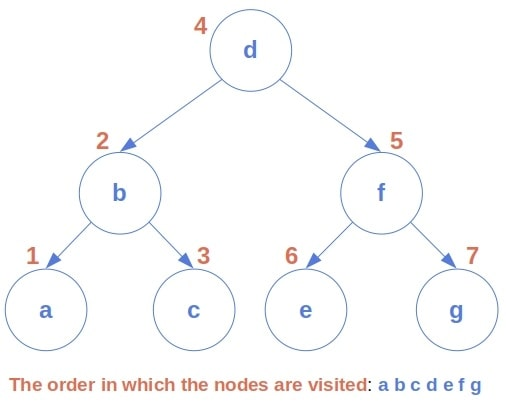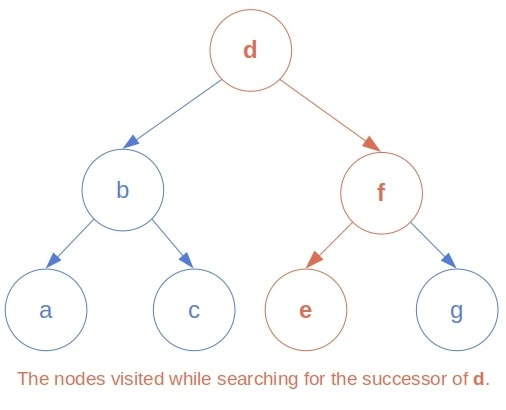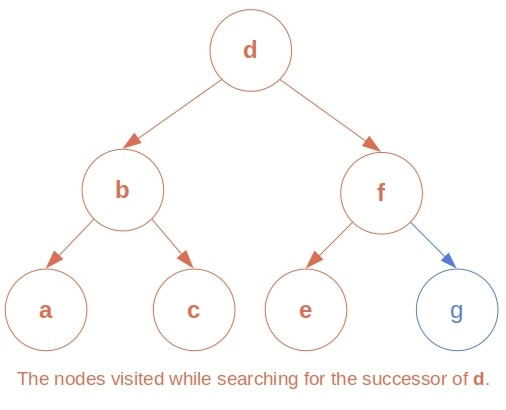## 1. Introduction

In this tutorial, we’ll show three ways to find the in-order successor of a node in a binary search tree (BST). In such a tree, each node isthat the nodes in its left sub-tree andthan the nodes in its right sub-tree.

Nodes can be numbers, strings, tuples, in general, objects that we can compare to one another.

## 2. What Is the In-Order Successor of a Node?

In-order traversal is a tree traversal technique we recursively define as follows:Applied to a BST, it visits the nodes in the non-decreasing order, as in the example of the following tree:Our goal is to find the immediate successor of a given nodein the in-order traversal of a tree. The successor is the smallest node of all those greater thanin the tree.

### 2.1. Where to Find the In-Order Successor?

If we take a look at the above image, we’ll see a pattern.

If a nodehas a right child, its successor is the minimal node of its right sub-tree. In other words, the node’s successor is the leftmost descendant of its right child in this case. Why? Because, after visiting, the traversal procedure will process the right sub-tree of, and the first to visit there is its leftmost leaf.

Ifhas no right child, its in-order successor is located above it in the tree, among its ancestors. While unfolding the recursive calls, the in-order traversal function will first visit the node whose left child was the most recent input. So, the‘s successor is the parent of the‘s youngest ancestor that is a left child.

## 3. Finding the Successor in an Out-Tree

Usually, we implement the nodes as structures with three attributes. One is the node’s content: the object placed at that place in the tree hierarchy. The other two are pointers to the node’s left and right children. Conceptually, this implementation corresponds to an out-tree: a tree with edges oriented away from its root.

### 3.1. Algorithm

To find a node’s in-order successor in an out-tree, we should first locate the node, memorizing the parents of the left children along the way. Once we find it, we check if it has the right child. If that’s the case, we return the right sub-tree’s leftmost leaf. Otherwise, we return the most recently memorized parent above the node:Ifis the maximal node, it has no successor. So, in that case, we return.

### 3.2. Complexity Analysis

Letbe the height of the whole BST. Letbe the length of the path fromtoin the tree. Ifhas no right child, we visitnodes to find. Afterward, we output the successor without further processing. Ifdoes have the right child, we find the successor upon reaching a leaf. The depth of any leaf in a tree is bounded from above by the tree’s height. So, we don’t make more thansteps in the worst case:If the tree is balanced, it will hold that, so our algorithm will run in logarithmic time, whereis the number of nodes in the tree. However, non-balanced trees may be degenerate. Their height isin the worst case, so the algorithm will be linear for such inputs.

## 4. Finding the Successor in a Bidirectional Implementation of BST

Usually, the nodes contain pointers only to their children. However, we sometimes store the pointers to parents as well. The reason is that it simplifies many tree operations. Even though we take more memory this way, the overhead is negligible most of the time.

### 4.1. Algorithm

In such a tree, we don’t have to keep track of the most recent parent with a left child while searching for. Instead, ifdoesn’t exist, we follow the to-parent pointers until we find the one we seek:The complexity of this approach is the same as that of the algorithm for out-trees.

The third and final way we’ll present is the in-order search. Since we’re looking for the immediate successor of, we can run the in-order traversal until visiting a node greater than. Since the in-order procedure visits the nodes in the non-descending order, we can be sure that the first visited node greater thanis its successor.

Here’s the pseudocode:### 5.1. Complexity Analysis

This method traversesnodes, whereis the rank ofamong the nodes in the tree:In the worst case,will be the greatest node, so. Therefore, the in-order search visits all thenodes and runs intime in the worst-case scenario.

## 6. Discussion

Which algorithm to choose? The first two are unaffected by the node’s rank, while the third approach’s complexity doesn’t depend on the tree’s height.

At first glance, it may seem that the first two algorithms are better. After all, if a tree is balanced, both are of logarithmic time complexity, whereas the in-order approach is linear in any case. However, building and maintaining a balanced tree isn’t a simple job. For example, if we often update the tree but mostly ask for the successor of a low-rank node, the in-order search will run faster in practice. The reason is that the updates won’t require rebalancing the tree.

What about duplicate entries? The first two algorithms locate the closest-to-the root node that contains. All the other nodes equal to it are in its left sub-tree, which we don’t process at all. So, the returned successor is going to be the first greater than. That is also the case with the third approach.

## 7. Conclusion

In this article, we presented three ways to find the in-order successor of a node. One is a simple extension of the in-order traversal algorithm. The other use of the fact is that a node’s successor is either the leftmost descendant of its right child or the parent of its youngest ancestor that is a left child.